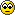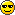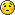# subnet

Can you explain for which reason
172.16.0.0/25
has 510 subnet and 126 host fo each subnet
The first is 172.16.0.128 and the others
11111111.11111111.11111111.10000000
How can i find the next network id
EXAMPle 172.16.0.128/25 172.16.0.254/25
and the other 172.16.0.?????
IS right 255.255.255.128
Thank you• I'm a bit confused by your question. Are you having difficulty understanding the idea of "stealing bits" from octets? Is this part of a bigger question?

If I see 172.16.0.0/25

My first host address would be 172.16.0.1
My last host address would be 172.16.0.127

My counter is 128 (256-12Does this make sense? It's all about stealing the bits to get to /25. You can find more about subnetting at www.learntosubnet.com
Pavlov
A+, Net+, i-Net+, CIW-A
MCP NT4, MCSA 2K, MCSE 2K
• Excuse me for my English Is not my mother tongue...Is it right that the subnet should be 510 and
126 hosts for subnet?
If i have a 172.16.0.0/25 network id
Can you tell me the first valid network id range
such us
172.16.0.128/25
Which is the first five network id 172.16.0.???/25
It will be 172.16.0.254/25??
Thank you and forgive me for my language
• based on my previous post - I would say your first 5 valid IP addresses are:
172.16.0.1
172.16.0.2
172.16.0.3
172.16.0.4
172.16.0.5

Last 5 would be:
172.16.0.123
172.16.0.124
172.16.0.125
172.16.0.126
172.16.0.127

/25 just indicates that the mask won't be the default of 255.255.255.0 - the /25 forces you to steal one bit from the next octet making your subnet mask 255.255.255.128.

Am I making sense to you - I think I may be adding to confusion.

Let me know if this helps.
Pavlov
A+, Net+, i-Net+, CIW-A
MCP NT4, MCSA 2K, MCSE 2K
think.
I was confused because I thought that the 172.16.0.0/25 was in this way

11111111.11111111.11111111.10000000
This rapresentation confused me because it is no possible to have 510 subnet if the third octet
was all 1
From your answer I see that the range of network id is however to find in a 172.16.0.0
However the question that I i finally find was

What are the first 10 possible subnets for this subnetted network:172.16.0.0/25
The first network id is 172.16.0.128/25 and there are 2^9-2 possible subnets
What are the ranges of valid host address for the first few subnets?
I don't remember where i see it.
Your explanation was very cleared don't worrythank you
• p.provino wrote:
Can you explain for which reason
172.16.0.0/25
has 510 subnet and 126 host fo each subnet
The first is 172.16.0.128 and the others
11111111.11111111.11111111.10000000
How can i find the next network id
EXAMPle 172.16.0.128/25 172.16.0.254/25
and the other 172.16.0.?????
IS right 255.255.255.128
Thank youWant the easy way?
11111111.11111111.11111111.10000000

0000000 = 7 zeros.
2^7 = 128.
128 - 2 = 126 hosts.

11111111.1 = 9 ones.
2^9 = 514
514 - 2 = 512 subnets.

Voilà!

0000000 = 7 zeros.
2^7 = 128.
128 - 2 = 126 hosts. -- why are you subtracting 2 ?

also:
11111111.1 = 9 ones.
2^9 = 514 --2^9 = 512, NOT 514
514 - 2 = 512 subnets.

FINALLY:
if its 172.16.0.0/16 does that mean that there are 65536 - 2 = 65534 subnets and 65534 hosts?

Thank you
• Member Posts: 28 ■□□□□□□□□□
I believe he's subtracting 192.168.0.0 which is the network id and 192.168.0.255 which is the broadcast id. Neither of which can be used for host IPs.
And we're using windows why?
• hmm... makes me wonder how you fished for this 'old' post.
Exquisite as a lily, illustrious as a full moon,
Magnanimous as the ocean, persistent as time.
• Member Posts: 28 ■□□□□□□□□□
I'm laid off.. got lots of timeAnd we're using windows why?